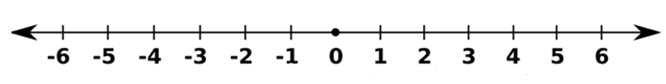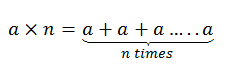# Properties of Multiplication of Integers

The multiplication of integers refers to the product of two or more integers. To recall, the set of numbers which consist of natural numbers, the additive inverse of natural numbers and zero are known as integers. Thus, integers can either be positive or negative and they have a magnitude and a sign associated with them. They are represented using Z or I. In this article, let us discuss the multiplication operations on integers and the properties of multiplication in detail.

## What are Integers?

Integers are the whole numbers but it does not include fractions. The integer can be either positive integer or negative integer.

For example: -53, 0, 1237, 31, -102, -401, -355, 86600 etc.

Integers can be located on the real number line as shown below.The four basic mathematical operations i.e. Addition, subtraction, multiplication, division and the properties related to these operations can be applied to integers as well.

## Multiplication of Integers

Multiplication is basically repeated addition. Therefore multiplication of integers is the repeated addition as:Where a and n are both integers.

## Properties of Multiplication of Integers

The properties of multiplication of integers are:

• Closure property
• Commutative property
• Multiplication by zero
• Multiplicative identity
• Associative property
• Distributive property

### Closure Property

According to this property, if two integers a and b are multiplied then their resultant a × b is also an integer. Therefore, integers are closed under multiplication.

a × b is an integer, for every integer a and b

### Commutative Property

The commutative property of multiplication of integers states that altering the order of operands or the integers does not affect the result of the multiplication.

a × b = b × a, for every integer a and b

### Multiplication by zero

On multiplying any integer by zero the result is always zero. In general, if a and b are two integers then,

a × 0 = 0 × a = 0

### Multiplicative Identity of Integers

On multiplying any integer by 1 the result obtained is the integer itself. In general, if a and b are two integers then,

a × 1 = 1 × a = a

Therefore 1 is the Multiplicative Identity of Integers.

### Associative Property

The result of the product of three or more integers is irrespective of the grouping of these integers. In general, if a, b and c are three integers then,

a × (b × c) = (a × b) × c

### Distributive Property

According to the distributive property of multiplication of integers, if a, b and c are three integers then,

a× (b + c) = (a × b) + (a × c)Keep visiting BYJU’S and Subscribe to our YouTube channel for learning number system and other mathematical topics in a fun and engaging way. Also, register now to get access to 1000+ hours of engaging video content.

1. Dozon Buron